International
Tables for
Crystallography
Volume B
Reciprocal space
Edited by U. Shmueli

International Tables for Crystallography (2010). Vol. B, ch. 2.2, pp. 223-224   | 1 | 2 |

## Section 2.2.5.3. Triplet relationships

C. Giacovazzoa*

aDipartimento Geomineralogico, Campus Universitario, 70125 Bari, Italy, and Institute of Crystallography, Via G. Amendola, 122/O, 70125 Bari, Italy
Correspondence e-mail: carmelo.giacovazzo@ic.cnr.it

#### 2.2.5.3. Triplet relationships

| top | pdf |

The basic formula for the estimation of the triplet phasegiven the parameter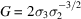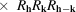is Cochran's (1955) formulawhere,is the atomic number of the jth atom andis the modified Bessel function of order n. In Fig. 2.2.5.1the distributionis shown for different values of G.Figure 2.2.5.1 | top | pdf |Curves of (2.2.5.6)for some values of.

The conditional probability distribution for, given a set ofand, is given (Karle & Hauptman, 1956; Karle & Karle, 1966) bywhere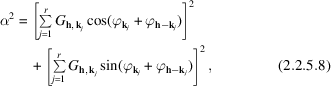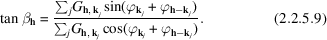is the most probable value for. The variance ofmay be obtained from (2.2.5.7)and is given bywhich is plotted in Fig. 2.2.5.2.Figure 2.2.5.2 | top | pdf |Variance (in square radians) as a function of α.

Equation (2.2.5.9)is the so-called tangent formula. According to (2.2.5.10), the larger is α the more reliable is the relation.

For an equal-atom structure.

The basic conditional formula for sign determination ofin cs. crystals is Cochran & Woolfson's (1955) formulawhereis the probability thatis positive and k ranges over the set of known values. The larger the absolute value of the argument of tanh, the more reliable is the phase indication.

An auxiliary formula exploiting all the's in reciprocal space in order to estimate a single Φ is the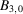formula (Hauptman & Karle, 1958; Karle & Hauptman, 1958) given bywhere C is a constant which differs for cs. and ncs. crystals,is the average value ofand p is normally chosen to be some small number. Several modifications of (2.2.5.12)have been proposed (Hauptman, 1964, 1970; Karle, 1970a; Giacovazzo, 1977b).

A recent formula (Cascarano, Giacovazzo, Camalli et al., 1984) exploits information contained within the second representation of Φ, that is to say, within the collection of special quintets (see Section 2.2.5.6):where k is a free vector. The formula retains the same algebraic form as (2.2.5.6), butwhere,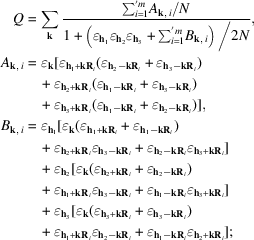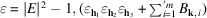is assumed to be zero if it is experimentally negative. The prime to the summation warns the reader that precautions have to be taken in order to avoid duplications in the contributions.

G may be positive or negative. In particular, if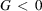the triplet is estimated negative.

The accuracy with which the value of Φ is estimated strongly depends on. Thus, in practice, only a subset of reciprocal space (the reflections k with large values of) may be used for estimating Φ.

(2.2.5.13)proved to be quite useful in practice. Positive triplet cosines are ranked in order of reliability by (2.2.5.13)markedly better than by Cochran's parameters. Negative estimated triplet cosines may be excluded from the phasing process and may be used as a figure of merit for finding the correct solution in a multisolution procedure.

### References

Cascarano, G., Giacovazzo, C., Camalli, M., Spagna, R., Burla, M. C., Nunzi, A. & Polidori, G. (1984). The method of representations of structure seminvariants. The strengthening of triplet relationships. Acta Cryst. A40, 278–283.Google Scholar
Cochran, W. (1955). Relations between the phases of structure factors. Acta Cryst. 8, 473–478.Google Scholar
Cochran, W. & Woolfson, M. M. (1955). The theory of sign relations between structure factors. Acta Cryst. 8, 1–12.Google Scholar
Giacovazzo, C. (1977b). Strengthening of the triplet relationships. II. A new probabilistic approach in. Acta Cryst. A33, 527–531.Google Scholar
Hauptman, H. (1964). The role of molecular structure in the direct determination of phase. Acta Cryst. 17, 1421–1433.Google Scholar
Hauptman, H. (1970). Communication at New Orleans Meeting of Am. Crystallogr. Assoc.Google Scholar
Hauptman, H. & Karle, J. (1958). Phase determination from new joint probability distributions: space group. Acta Cryst. 11, 149–157.Google Scholar
Karle, J. (1970a). An alternative form for B3.0, a phase determining formula. Acta Cryst. B26, 1614–1617.Google Scholar
Karle, J. & Hauptman, H. (1956). A theory of phase determination for the four types of non-centrosymmetric space groups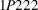,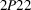,,. Acta Cryst. 9, 635–651.Google Scholar
Karle, J. & Hauptman, H. (1958). Phase determination from new joint probability distributions: space group. Acta Cryst. 11, 264–269.Google Scholar
Karle, J. & Karle, I. L. (1966). The symbolic addition procedure for phase determination for centrosymmetric and non-centrosymmetric crystals. Acta Cryst. 21, 849–859.Google Scholar